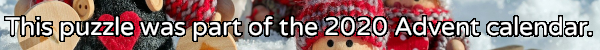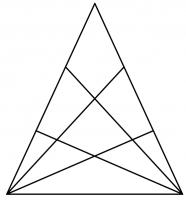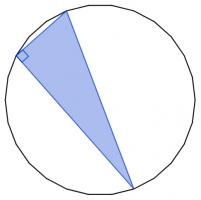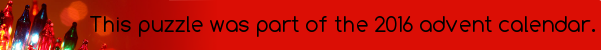mscroggs.co.uk
mscroggs.co.uksubscribe

# Puzzles

## 19 DecemberThe diagram to the right shows a triangle. Two of the sides of the triangle have been split into three pieces, with lines drawn from the opposite vertex. In total, the diagram now contains 27 triangles of any size.
Another triangle has two of its sides split into eight pieces, with lines drawn from the opposite vertex. How many triangles (of any size) would this create?

## 5 December28 points are spaced equally around the circumference of a circle. There are 3276 ways to pick three of these points. The three picked points can be connected to form a triangle. Today's number is the number of these triangles that are isosceles.

## 2 DecemberYou have 15 sticks of length 1cm, 2cm, ..., 15cm (one of each length). How many triangles can you make by picking three sticks and joining their ends?
Note: Three sticks (eg 1, 2 and 3) lying on top of each other does not count as a triangle.
Note: Rotations and reflections are counted as the same triangle.

## 12 DecemberThere are 2600 different ways to pick three vertices of a regular 26-sided shape. Sometimes the three vertices you pick form a right angled triangle.These three vertices form a right angled triangle.
Today's number is the number of different ways to pick three vertices of a regular 26-sided shape so that the three vertices make a right angled triangle.

## Is it equilateral?

In the diagram below, $$ABDC$$ is a square. Angles $$ACE$$ and $$BDE$$ are both 75°.
Is triangle $$ABE$$ equilateral? Why/why not?

## 20 DecemberEarlier this year, I wrote a blog post about different ways to prove Pythagoras' theorem. Today's puzzle uses Pythagoras' theorem.
Start with a line of length 2. Draw a line of length 17 perpendicular to it. Connect the ends to make a right-angled triangle. The length of the hypotenuse of this triangle will be a non-integer.
Draw a line of length 17 perpendicular to the hypotenuse and make another right-angled triangle. Again the new hypotenuse will have a non-integer length. Repeat this until you get a hypotenuse of integer length. What is the length of this hypotenuse?

## Cutting corners

The diagram below shows a triangle $$ABC$$. The line $$CE$$ is perpendicular to $$AB$$ and the line $$AD$$ is perpedicular to $$BC$$.
The side $$AC$$ is 6.5cm long and the lines $$CE$$ and $$AD$$ are 5.6cm and 6.0cm respectively.
How long are the other two sides of the triangle?

## Two triangles

Source: Maths Jam
The three sides of this triangle have been split into three equal parts and three lines have been added.
What is the area of the smaller blue triangle as a fraction of the area of the original large triangle?

## Archive

Show me a random puzzle
▼ show ▼